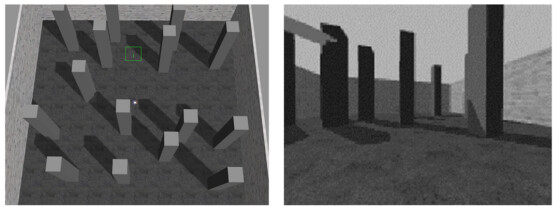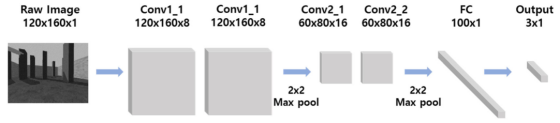# Visual obstacle avoidance of UNMANNED aerial vehicles through sequence neural network imitation learning

Obstacle avoidance is considered to be one of the most basic requirements in addition to path planning or following ability for agents that are fully capable of autonomous navigation in a given environment. An end-to-end imitative learning framework is proposed for vision-based obstacle avoidance techniques that can be applied to uAVs where such decision strategies are trained based on supervision of actual human flight data. We use deep learning to map visual input to steering direction. After successfully training the neural network, the drone should be able to mimic the decisions of human experts, using only visual input to avoid obstacles during autonomous navigation. The overall framework of the proposed sequence neural network imitation learning based on human data is shown in figure.1. Training data collection and pretreatment

Gazebo simulator is used to provide simulation environment and training data for neural network. A square map with walls around the boundary was designed, with random obstacles placed throughout the map, and the rotor firefly model was used to obtain training data. A sample image of the simulation environment and drone camera is shown in Figure 2 below. Human expert flight data in the simulated environment was recorded, meaning that images of each time step and corresponding control inputs from the expert were aggregated into the training data set.Figure 2 Example of simulation environment (left) and UAV image (right)

1. Four types of neural network architecture
2. Fully connected Neural Network (FCNN)

The architecture of the FCNN model used for comparison in this study is shown in figure. FCNN uses three fully connected layers, each with 100 nodes, and the input images are flattened into (120×160) (120×160) vectors of the neural network. The rectifying linear unit (ReLU) function is used as the activation function for each hidden layer. The final output of the neural network is the (3×1) (3×1) vector, where the largest element of the output vector is considered to be the predicted value of the neural network.2. Convolutional Neural Network (CNN)

The CNN architecture used in this study is shown in the figure. Two sets of double convolutional layers are used, where the second set is followed by the maximum pooling operation of the first set. A (3×3) (3×3) convolution filter with step size 1 and a (2×2)(2×2) maximum pooling kernel are used for two convolution sets. Finally, the output of the convolution layer is flattened into the (100×1) (100×1) pooled vector, and then the output layer.1. Recursive Neural Network (RNN)

Since vision-based obstacle avoidance can be considered as a sequential task, RNN was used in this study. Instead of using the original image as the input to the RNN, features extracted from the image are used as the input to the RNN. This is accomplished through the concept of transfer learning, in which fragments of a pre-trained neural network are used as feature extractors for another neural network. The network is mainly composed of two parts: a pre-trained feature extraction CNN in the front, and a gated loop unit (GRU) RNN in the back. In general, the long and short term memory (LSTM) unit is one of the most widely used units to implement the ability of RNN to deal with gradient extinction problems.

Before the RNN training process, feature extraction CNN is trained on CIFAR-10 data set, and the bottom layer is used as the feature extractor, because IT is known that CNN learns more general features at the bottom layer. Later, in the TRAINING stage of RNN, the weight and deviation of CNN are fixed, and only the parameters of RNN are updated. The architecture of the RNN model is shown in figure 1.4. 3D Convolutional Neural Network (3D-CNN)
The 3D-CNN architecture used in this framework is composed of four convolution layers. The architecture of the 3D-CNN model is shown in Figure 7. As an alternative to the convolution layer with a 2d convolution filter, a 3D filter is applied to a pile of continuous images. Taking time as an extra physical dimension, the extra dimension in the convolution filter allows 3D-CNN to obtain the spatio-temporal features in a given data.

1 Like

The obstacle avoidance function in indoor or narrow spaces on the market is not ideal
But this direction is worth digging deeper